# Math Happens When Two of 1632’s Factors Look in a Mirror!

### Today’s Puzzle:

Both 12 and 102 are factors of 1632. Something special happens when either one squares itself and looks in a mirror. Solving this puzzle from Math Happens will show you what happens to 12 and 12².

You can see that puzzle on page 33 of this e-edition or this pdf of the Austin Chronicle. You can find other Math Happens Puzzles here.

This next puzzle will help you discover what happens when 102 and 102² look in a mirror!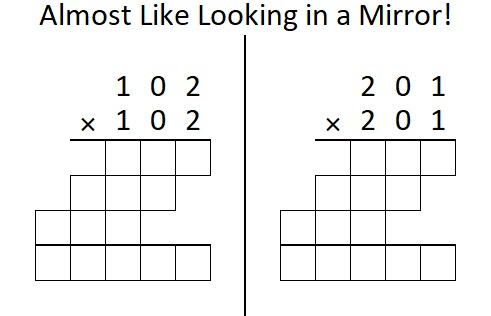Why do you suppose the squares of (12, 21) and (102, 201) have that mirror-like property?

### Factor Trees for 1632:

There are many possible factor trees for 1632, but today I will focus on two trees that use factor pairs containing either 12 or 102:### Factors of 1632:

• 1632 is a composite number.
• Prime factorization: 1632 = 2 × 2 × 2 × 2 × 2 × 3 × 17, which can be written 1632 = 2⁵ × 3 × 17.
• 1632 has at least one exponent greater than 1 in its prime factorization so √1632 can be simplified. Taking the factor pair from the factor pair table below with the largest square number factor, we get √1632 = (√16)(√102) = 4√102.
• The exponents in the prime factorization are 5, 1, and 1. Adding one to each exponent and multiplying we get (5 + 1)(1 + 1)(1 + 1) = 6 × 2 × 2 = 24. Therefore 1632 has exactly 24 factors.
• The factors of 1632 are outlined with their factor pair partners in the graphic below.### More about the Number 1632:

1632 is the hypotenuse of a Pythagorean triple:
768-1440-1632, which is (8-15-17) times 96.

1632 is the difference of two squares in EIGHT different ways:
409² – 407² = 1632,
206² – 202² = 1632,
139² – 133² = 1632,
106² – 98² = 1632,
74² – 62² = 1632,
59² – 43² = 1632,
46² – 22² = 1632, and
41² – 7² = 1632.

That last difference of two squares means 1632 is only 49 numbers away from the next perfect square, 1681.

# Math Happens When Factors of 1620 Make Sum-Difference

### Math Happens Puzzle:

Math Happens put another one of my puzzles in the Austin Chronicle and the Orange Leader!

You can see the puzzle on page 23 of this e-edition or this pdf of the newspaper. You can also find links to all of my Sum-Difference puzzles here.

Here is another one of Math Happen’s amazing puzzles. The tabletops shown are exactly the same. Click on it to see proof!

Math Happens in many different ways as you can see in their blog post from February 5. You can also look for Math Happens on a page in the middle of each of these  2020 issues or 2021 issues of the Austin Chronicle newspaper online.

Would you like puzzles like these in your community newspaper? Have your paper contact Math Happens on Twitter and make it happen!

### Today’s Sum-Difference Puzzles:

Just like the number 6 in the newspaper puzzle above, 180 and 1620 both have factor pairs that make sum-difference. To help you solve these puzzles, I’ve listed all of their factor pairs in the graphics below the puzzle.As shown below, 180 has nine factor pairs. One of those pairs adds up to 41, and another one subtracts to 41. Put the factors in the appropriate boxes in the first puzzle.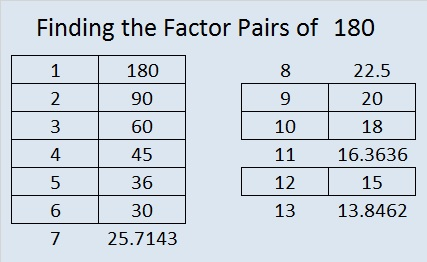The needed factors for the second puzzle are multiples of the numbers in the first puzzle. 1620 has fifteen factor pairs. One of the factor pairs adds up to ­123, and a different one subtracts to 123. If you can identify those factor pairs, then you can solve the second puzzle!

### What Are the Factors of 1620?• 1620 is a composite number.
• Prime factorization: 1620 = 2 × 2 × 3 × 3 × 3 × 3 × 5, which can be written 1620 = 2² × 3⁴ × 5.
• 1620 has at least one exponent greater than 1 in its prime factorization so √1620 can be simplified. Taking the factor pair from the factor pair table below with the largest square number factor, we get √1620 = (√324)(√5) = 18√5.
• The exponents in the prime factorization are 4, 2 and 1. Adding one to each exponent and multiplying we get (2 + 1)(4 + 1)(1 + 1) = 3 × 5 × 2 = 30. Therefore 1620 has exactly 30 factors.
• The factors of 1620 are outlined with their factor pair partners in the graphic above.

### Factor Trees for 1620:

Here are two of the MANY possible factor trees for 1620: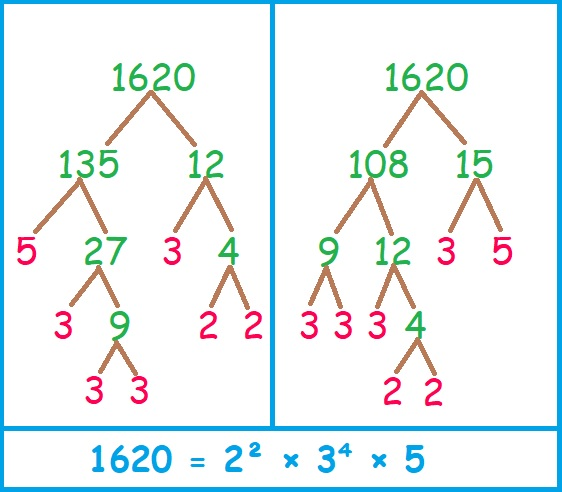Hint: I chose to build these factor trees with those two sets of factor pairs for a reason.

### More about the Number 1620:

1620 is the sum of the interior angles of a hendecagon (11-sided polygon) because
(11 – 2)180 = 1620.1620 is the sum of two squares:
36² + 18² = 1620.

1620 is the hypotenuse of a Pythagorean triple:
972-1296-1620, calculated from 36² – 18², 2(36)(18), 36² + 18².
It is also (3-4-5) times 324.

This is only some of the math that happens with the number 1620.

# 1600 How Would You Describe This Horse Race?

### Today’s Puzzle:

Do most of the numbers from 1501 to 1600 have 2 factors, 4 factors, 6 factors, or what? A horse race is a fun way to find the answer to that puzzle!

As I’ve done several times before, I’ve made a horse race for this multiple of 100 and the 99 numbers before it. A horse moves when a number comes up with a particular amount of factors. Some of the races I’ve done in the past have been exciting with several lead changes. In other races, one horse ran quite quickly, leaving all other horses in the dust. One previous horse race resulted in a tie.

How will you describe this horse race? Exciting or boring? Surprizing or predictable? Pick your pony and watch the race to the end before you decide on an adjective.

Click here if you would like the Horse Race to be slightly bigger.make science GIFs like this at MakeaGif
Wow! I’ve not seen that happen before! Visually it looks like 4 won the race, but this horse race is really about finding the mode There are two modes, 4 and 8, for the amount of factors for the numbers from 1501 to 1600. It’s about which amount of factors comes up most often for the entire set of numbers, not which one of those occurs first. Thus, for that reason, I would describe the horse race above as deceptive. That horse race looked at the amount of factors five numbers at a time. Here’s what happens if we look at ten numbers at a time:
In the second horse race, it is much more clear that the race ends in a tie, and the mode is BOTH 4 and 8.

### Prime Factorization of Numbers from 1501 to 1600:Of those 100 numbers, 38 have square roots that can be simplified; 62 do not.

### Factor Trees for 1600:

1600 has MANY possible factor trees. Some are symmetrical; some are not. Here are two nicely shaped ones:### Factors of 1600:

• 1600 is a composite number and a perfect square.
• Prime factorization: 1600 = 2 × 2 × 2 × 2 × 2 × 2 × 5 × 5, which can be written 1600 = 2⁶ × 5².
• 1600 has at least one exponent greater than 1 in its prime factorization so √1600 can be simplified. Taking the factor pair from the factor pair table below with the same number for both factors, we get
√1600 = (√40)(√40) = 40. However, you could also use
√1600 = (√4)(√400) = 2 × 20 = 40,
√1600 = (√16)(√100) = 4 × 10 = 40, or
√1600 = (√25)(√64) = 5 × 8 = 40.
• The exponents in the prime factorization are 6 and 2. Adding one to each exponent and multiplying we get (6 + 1)(2 + 1) = 7 × 3 = 21. Therefore 1600 has exactly 21 factors.
• The factors of 1600 are outlined with their factor pair partners in the graphic below.### More about the Number 1600:

1600 is the sum of two squares:
32² + 24² = 1600.

1600 is the hypotenuse of two Pythagorean triples:
448-1536-1600, calculated from 32² – 24², 2(32)(24), 32² + 24².
It is also (7-24-25) times 64.
960-1280-1600, which is (3-4-5) times 320.

1600 looks square in some other bases:
1600 = 1(40²) + 0(40) + 0(1), so it’s 100₄₀.
1600 =1(39²) + 2(39) + 1(1), so it’s 121₃₉.
1600 =1(38²) + 4(38) + 4(1), so it’s 144₃₈.
1600 =1(37²) + 6(37) + 9(1), so it’s 169₃₇.

Furthermore, 1600 is a repdigit in base 7:
1600 = 4(7³ + 7² + 7¹ + 7º), so it’s 4444₇.

# 1590 A Single Rose

### Today’s Puzzle:

A single rose can be an elegant expression of affection. This single rose is a level 5 puzzle. Can you find its factors?Here’s the same puzzle without any added color:### Two Factor Trees for 1590:

There are several possible factor trees for 1590. Here are two of them.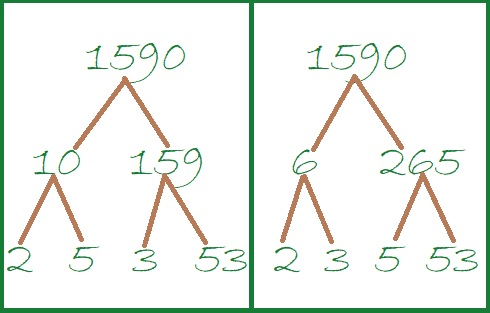### Factors of 1590:

• 1590 is a composite number.
• Prime factorization: 1590 = 2 × 3 × 5 × 53.
• 1590 has no exponents greater than 1 in its prime factorization, so √1590 cannot be simplified.
• The exponents in the prime factorization are 1, 1, 1, and 1. Adding one to each exponent and multiplying we get (1 + 1)(1 + 1)(1 + 1)(1 + 1) = 2 × 2 × 2 × 2 = 16. Therefore 1590 has exactly 16 factors.
• The factors of 1590 are outlined with their factor pair partners in the graphic below.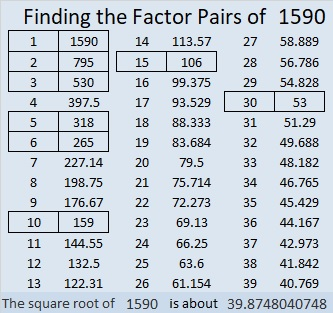### More about the Number 1590:

1590 is the hypotenuse of FOUR Pythagorean triples:
138-1584-1590, which is 6 times (23-264-265),
576-1482-1590, which is 6 times (96-247-265)
840-1350-1590, which is 30 times (28-45-53),
954-1272-1590, which is (3-4-5) times 318.

# 1564 Two Candles

### Today’s Puzzle:

Candles that are lit in the darkness can be seen from quite a distance away.  Candles and candlelight are symbols of Christmas. The babe born on that first Christmas day would become the Light of the World.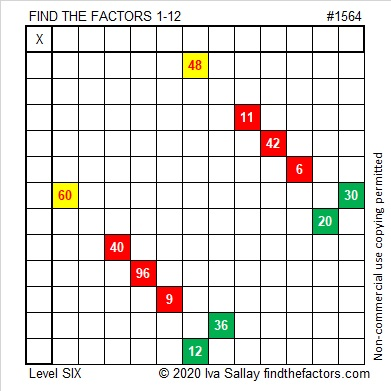What makes a level 6 puzzle more difficult? Can you see that the common factor of 60 and 30 might be 5, 6, or 10? Which one should you use? The other two won’t work with the other clues in the puzzle.

Likewise, the common factor of 48 and 12 might be 4, 6, or 12. Don’t guess which one to use! Use logic, and find the solution to this puzzle.

One blank row and one blank column intersect in a single cell. Can you determine what number belongs in that cell before you write any other factors? That is the first thing I would do.

Here is the same puzzle without any added color: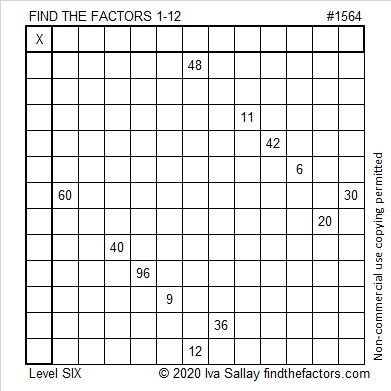### Factor Tree for 1564:

64 is divisible by 4, so 1564 is also. Here is a factor tree for 1564 that divisibility fact:### Factors of 1564:

• 1564 is a composite number.
• Prime factorization: 1564 = 2 × 2 × 17 × 23, which can be written 1564 = 2² × 17 × 23.
• 1564 has at least one exponent greater than 1 in its prime factorization so √1564 can be simplified. Taking the factor pair from the factor pair table below with the largest square number factor, we get √1564 = (√4)(√391) = 2√391.
• The exponents in the prime factorization are 2, 1, and 1. Adding one to each exponent and multiplying we get (2 + 1)(1 + 1)(1 + 1) = 3 × 2 × 2 = 12. Therefore 1564 has exactly 12 factors.
• The factors of 1564 are outlined with their factor pair partners in the graphic below.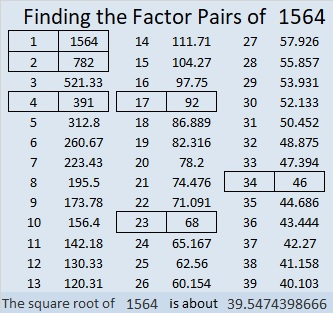### More about the Number 1564:

1564 is the hypotenuse of a Pythagorean triple:
736-1380-1564, which is (8-15-17) times 92.

1564 is the difference of two squares in two different ways:
392² – 390² = 1564, and
40² – 6² = 1564. That means we are only 36 numbers away from 40² = 1600.

1564 is in this cool pattern:# 1530 Jack-o’-lantern

### Today’s Puzzle:

Here’s a Jack-O’-Lantern Puzzle for you to enjoy. It’s a Level 5 puzzle so it might be more of a trick than a treat. Remember to use logic every step of the way instead of guessing and checking.Here’s the same puzzle without any added color:### Factors of 1530:

15 is half of 30, so 1530 is divisible by 6 just like all these numbers are divisible by 6: 12, 24, 36, 48, 510, 612, 714, 816, 918, 1020, 1122, 1224, 1326, 1428, and so forth.

• 1530 is a composite number.
• Prime factorization: 1530 = 2 × 3 × 3 × 5 × 17, which can be written 1530 = 2 × 3² × 5 × 17.
• 1530 has at least one exponent greater than 1 in its prime factorization so √1530 can be simplified. Taking the factor pair from the factor pair table below with the largest square number factor, we get √1530 = (√9)(√170) = 3√170.
• The exponents in the prime factorization are 1, 2, 1, and 1. Adding one to each exponent and multiplying we get (1 + 1)(2 + 1)(1 + 1)(1 + 1) = 2 × 3 × 2 × 2 = 24. Therefore 1530 has exactly 24 factors.
• The factors of 1530 are outlined with their factor pair partners in the graphic below.### More about the Number 1530:

51 × 30 = 1530. Did you notice that the same digits appear on both sides of the equal sign and only +, -, ×, ÷, (), or exponents were used to make a true statement? 1530 is only the 25th number that can make that claim, so we call it the 25th Friedman number.

There are MANY possible factor trees for 1530, but let’s celebrate that it is also a Friedman number with this one: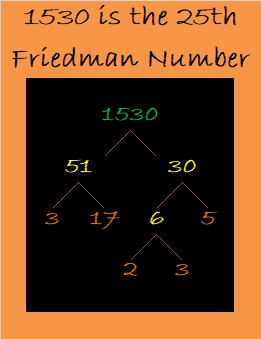1530 is the hypotenuse of FOUR Pythagorean triples:
234-1512-1530, which is 18 times (13-84-85),
648-1386-1530, which is 18 times (36-77-85),
720-1350-1530, which is (8-15-17) times 90, and
918-1224-1530, which is (3-4-5) times 306.

# 1512 Bigger Bites of Cake

### Using the Cake Method:

I like using the cake method to find the prime factorization of a number. I also use it to find square roots.

If you know the multiplication table well, dividing by any number from 2 to 9 is not difficult.

It is easy to check to see if a number is divisible by 4 or by 9. And it is actually easier to divide by 4 once than it is to divide by 2 twice or to divide by 9 once than it is to divide by 3 twice. That way we get to take bigger bites of cake!

I know that 1512 is divisible by 4 because the number formed from the last two digits in order, 12, is divisible by 4.

I also know that 1512 is divisible by 9 because 1 + 5 + 1 + 2 = 9.

Thus, I’ll begin by dividing first by 4 and then the result by 9 as illustrated below: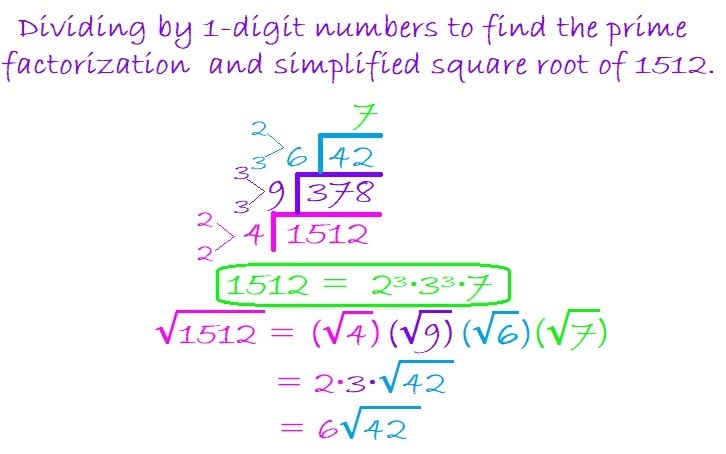When my new divisor becomes 42, if I didn’t remember where it appears in the multiplication table, I would still be fine. I know that 42 is divisible by 2 because it is even and 3 because 4 + 2 = 6, a number divisible by 3.

Thus I can divide 42 by 6 because 2 × 3 = 6, and it is far easier to divide 42 by 6 than it is to divide it first by 2 and then by 3.

To take the square root of 1512, I simply take the square root of the numbers on the outside of the cake. 4 and 9 are perfect squares so I use their square roots. Neither 6 nor 7 has any perfect squares, so I just multiply them together to get 42.

Dividing 1512 this way allowed me to make just a three-layer cake instead of a 6-layer cake to find its prime factorization and its square root!

Some people prefer to do yard work more than having cake, so here is one of the MANY possible factor trees for 1512. Make sure you pick up all seven of the leaves with prime numbers wherever they are.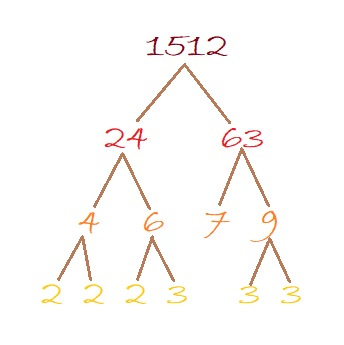### Factors of 1512:

• 1512 is a composite number.
• Prime factorization: 1512 = 2 × 2 × 2 × 3 × 3 × 3 × 7, which can be written 1512 = 2³ × 3³ × 7.
• 1512 has at least one exponent greater than 1 in its prime factorization so √1512 can be simplified. Taking the factor pair from the factor pair table below with the largest square number factor, we get √1512 = (√36)(√42) = 6√42.
• The exponents in the prime factorization are 2, 1, and 2. Adding one to each exponent and multiplying we get (3 + 1)(3 + 1)(1 + 1) = 4 × 4 × 2 = 32. Therefore 1512 has exactly 32 factors.
• The factors of 1512 are outlined with their factor pair partners in the graphic below.# 1470 Can You Find Factor Pairs That Make Sum-Difference?

### Today’s Puzzles:

I bet you can find a factor pair of 30 that adds up to 13 as well as another factor pair of 30 that subtracts to give you 13.

If you can solve that simple puzzle, then you will be able to solve the puzzle next to it. Even though 1470 has 12 different factor pairs, you don’t have to worry too much about them: All of the answers to the second puzzle are just _____ times the answers to the first puzzle! (And 1470 is _____² times 30.)Likewise, don’t get scared off with this next set of puzzles one of which wants you to find the factor pairs of 518616 that add up or subtract to 1470. Crazy, right? Again, if you can solve the first puzzle in the set, and if you can multiply a 3-digit number by a 1-digit number, you can easily solve the second puzzle because the answers are just _________ times the answers to the first puzzle in the set! (And 518616 is merely _________² times 6.)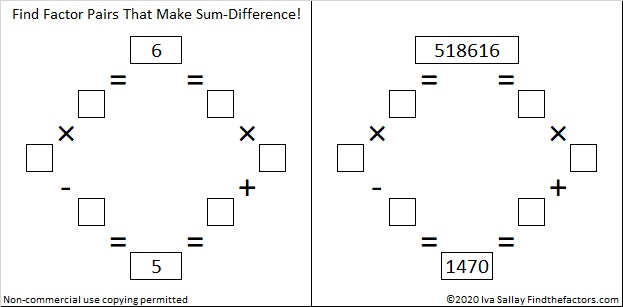If you need more help than what I’ve already said, scroll down to the factor trees for 1470. I selected those particular trees for a reason!

### Factors of 1470:

• 1470 is a composite number.
• Prime factorization: 1470 = 2 × 3 × 5 × 7 × 7, which can be written 1470 = 2 × 3 × 5 × 7²
• 1470 has at least one exponent greater than 1 in its prime factorization so √1470 can be simplified. Taking the factor pair from the factor pair table below with the largest square number factor, we get √1470 = (√49)(√30) = 7√30
• The exponents in the prime factorization are 1, 1, 1, and 2. Adding one to each exponent and multiplying we get (1 + 1)(1 + 1)(1 + 1)(2 + 1) = 2 × 2 × 2 × 3 = 24. Therefore 1470 has exactly 24 factors.
• The factors of 1470 are outlined with their factor pair partners in the graphic below.### A Few Factor Trees for 1470:### More Facts about the Number 1470:

1470 is the average of 14² and 14³. That simple fact makes 1470 the 14th Pentagonal Pyramidal Number.

1470 is the hypotenuse of a Pythagorean triple:
882-1176-1470 which is (3-4-5) times 294

Hmm… that same factor pair showed up again!

# 1452 Poinsettia Plant Mystery

### Today’s Puzzle:

Merry Christmas, Everybody!

The poinsettia plant has a reputation for being poisonous, but it has never been a part of a whodunnit, and it never will. Poinsettias actually aren’t poisonous.

Multiplication tables might also have a reputation for being deadly, but they aren’t either, except maybe this one. Can you use logic to solve this puzzle without it killing you?To solve the puzzle, you will need some multiplication facts that you probably DON’T have memorized. They can be found in the table below. Be careful! The more often a clue appears, the more trouble it can be:Notice that the number 60 appears EIGHT times in that table. Lucky for you, it doesn’t appear even once as a clue in today’s puzzle!

### Factors of 1452:

Now I’d like to factor the puzzle number, 1452. Here are a few facts about that number:

1 + 4 + 5 + 2 = 12, which is divisible by 3, so 1452 is divisible by 3.
1 – 4 + 5 – 2 = 0, which is divisible by 11, so 1452 is divisible by 11.

• 1452 is a composite number.
• Prime factorization: 1452 = 2 × 2 × 3 × 11 × 11, which can be written 1452 = 2² × 3 × 11²
• 1452 has at least one exponent greater than 1 in its prime factorization so √1452 can be simplified. Taking the factor pair from the factor pair table below with the largest square number factor, we get √1452 = (√484)(√3) = 22√3
• The exponents in the prime factorization are 2, 1, and 2. Adding one to each exponent and multiplying we get (2 + 1)(1 + 1)(2 + 1) = 3 × 2 × 3 = 18. Therefore 1452 has exactly 18 factors.
• The factors of 1452 are outlined with their factor pair partners in the graphic below.### 1452 Factor Tree:

To commemorate the season, here’s a factor tree for 1452:### More about the Number 1452:

1452 is the difference of two squares three different ways:
364² – 362² = 1452,
124² – 118² = 1452, and
44² – 22² = 1452.

Have a very happy holiday!

# 1449 Christmas Star

If you’ve ever wished you knew the multiplication table better, then make that wish upon this Christmas star. If you use logic and don’t give up,  then you can watch your wish come true!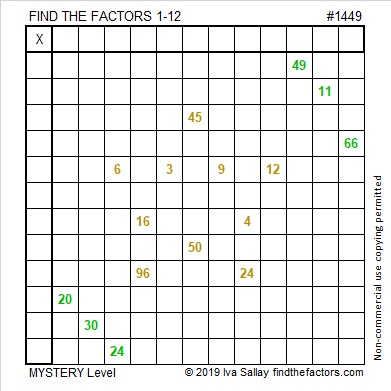I number the puzzles to distinguish them from one another. That star puzzle is way too big for a factor tree made with its puzzle number:Here’s more about the number 1449:

• Prime factorization: 1449 = 3 × 3 × 7 × 23, which can be written 1449 = 3² × 7 × 23
• 1449 has at least one exponent greater than 1 in its prime factorization so √1449 can be simplified. Taking the factor pair from the factor pair table below with the largest square number factor, we get √1449 = (√9)(√161) = 3√161
• The exponents in the prime factorization are 2, 1, and 1. Adding one to each exponent and multiplying we get (2 + 1)(1 + 1)(1 + 1) = 3 × 2 × 2 = 12. Therefore 1449 has exactly 12 factors.
• The factors of 1449 are outlined with their factor pair partners in the graphic below.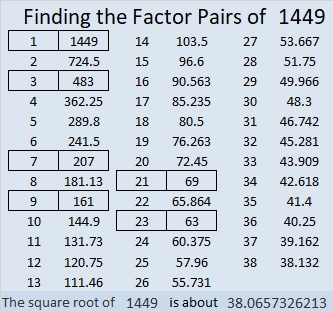1449 is the difference of two squares in 6 different ways:
725² – 724² = 1449
243² – 240² = 1449
107²-100² = 1449
85² – 76² = 1449
45² – 24² = 1449
43² – 20² = 1449